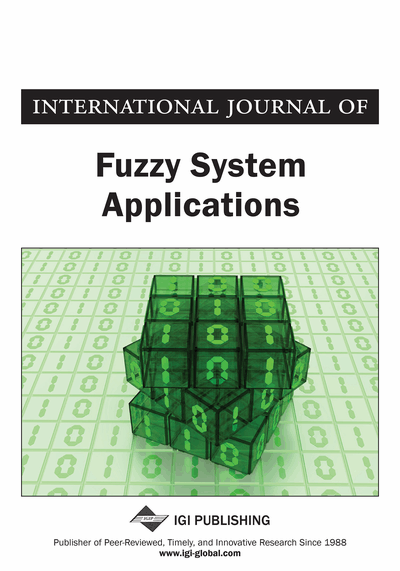# A Greedy Algorithm for Fuzzy Shortest Path Problem using Quasi-Gaussian Fuzzy Weights

Madhushi Verma (Department of Computer Engineering, IIT(BHU), Varanasi, India) and K. K. Shukla (Department of Computer Engineering, IIT(BHU), Varanasi, India)
DOI: 10.4018/ijfsa.2013040104

## Abstract

Several algorithms exist to determine the shortest path in a network for the crisp case where the weights are real numbers. In the real world, these weights represent parameters like cost, packet arrival time, link capacity etc which are not naturally precise. To model the uncertainty involved, for the first time we use the Gaussian fuzzy numbers as weights and a method has been presented in this paper to determine the fuzzy shortest path. Gaussian membership functions are preferred over other membership functions (triangular and trapezoidal) that are easy to analyze because it is continuous and differentiable enabling efficient gradient based optimization and it is simpler to represent because it requires fewer parameters. The issue of performing fuzzy arithmetic operations to calculate the fuzzy shortest path length and the corresponding fuzzy shortest path in the network has been addressed and to tackle it the concept of decomposed fuzzy numbers has been used. Also, a greedy algorithm which is an extension of Dijkstra’s algorithm for fuzzy shortest path problem has been proposed.
Article Preview
Top

## Introduction

In the field of mathematics and computer science, graphs are structures used to depict the pair wise relations between the objects from a collection. A graph can be represented aswhereis the set of vertices andis the set of edges connecting pair of vertices. In a network, a path is an alternating sequence of vertices and edges connecting a source node and a destination node. In general, there can be more than one path connecting the source node and the destination node, the shortest path is one where the sum of the weights assigned to the edges is minimum. The problem of finding the shortest path is of great importance in graph theory as it finds various real life applications like communication, computer networks, transport, routing, supply chain management etc. (Elizabeth & Sujatha, 2011; Hernandes et al., 2007; Ravishankar et al., 2012). An example of finding the shortest path could be to determine the route between city A and city B on a road map with minimum travel time. Here the various connecting cities between city A and city B can be represented as vertices and their connecting road segments can be represented as edges connecting the vertices. In the real world the weights assigned to these edges correspond to cost, time, capacities or demand which are represented as real numbers. However these parameters are not naturally precise and the uncertainty involved cannot be modelled using real numbers. This gives rise to the fuzzy shortest path problem (FSPP) where the weights are taken as fuzzy numbers in order to deal with the uncertainty. In a graph, various types of fuzziness are possible which are given by Blue et al (2002) as:

• Type I: Fuzzy set of crisp graphs;

• Type II: Crisp vertex set and fuzzy edge set;

• Type III: Crisp vertices and edges with fuzzy connectivity;

• Type IV: Fuzzy vertex set and crisp edge set;

• Type V: Crisp graph with fuzzy weights.

To tackle the non-statistical uncertainty in problems, fuzzy set theory was proposed by Zadeh (1978) and using this theory a lot of work has been done in the area of shortest path problem. Dubois & Prade (1980) first introduced the “Fuzzy Shortest Path Problem” and found the fuzzy shortest path length (FSPL) in a network using the Floyd’s algorithm and the Ford’s algorithm. However, the corresponding shortest path may not be present in the network. A dynamical programming recursion-based fuzzy algorithm was proposed by Klein (1991) and in 2006 another dynamic programming approach was used to solve FSPP using triangular fuzzy numbers (Kung et al., 2006). Later, an attempt was made to determine the fuzzy shortest path present in the network corresponding to the calculated FSPL using different fuzzy numbers (triangular, trapezoidal and discrete fuzzy numbers) as arc lengths and was successfully accomplished by using the concept of “Similarity Measure” where a comparison was made between each path length and the shortest path length, the one with the highest similarity degree was concluded as the fuzzy shortest path (FSP) (Chuang & Kung, 2005; Chuang & Kung, 2006; Sujatha & Elizabeth, 2011). Other than similarity measure, “ranking index” has also been used for determining the FSP in the network by comparing individual path lengths with the shortest path length and assigning ranks in order to determine the FSP (Elizabeth & Sujatha, 2011).

## Complete Article List

Search this Journal:
Reset
Open Access Articles: Forthcoming
Volume 9: 4 Issues (2020): Forthcoming, Available for Pre-Order
Volume 8: 4 Issues (2019)
Volume 7: 4 Issues (2018)
Volume 6: 4 Issues (2017)
Volume 5: 4 Issues (2016)
Volume 4: 4 Issues (2015)
Volume 3: 4 Issues (2013)
Volume 2: 4 Issues (2012)
Volume 1: 4 Issues (2011)
View Complete Journal Contents Listing